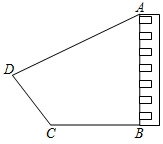(参考数据: $\sin 28^{\circ} \approx 0.47, \cos 28^{\circ} \approx 0.88, \tan 28^{\circ} \approx 0.53$ )$\text{A.}$ $76.9 \mathrm{~m}$ $\text{B.}$ $82.1 \mathrm{~m}$ $\text{C.}$ $94.8 \mathrm{~m}$ $\text{D.}$ $112.6 \mathrm{~m}$#### 解析：

①点击 首页查看更多试卷和试题 , 点击查看 本题所在试卷